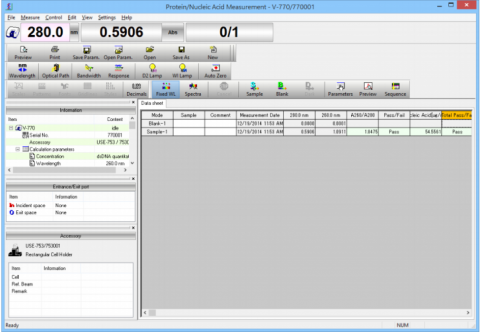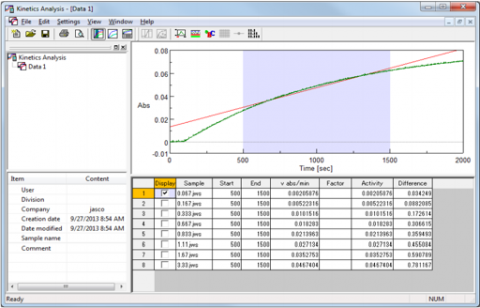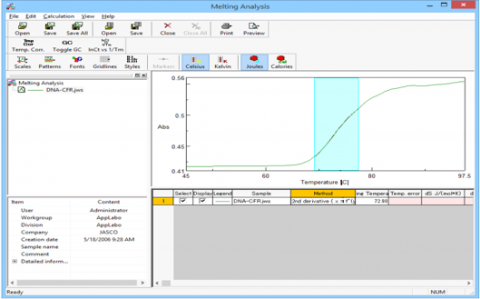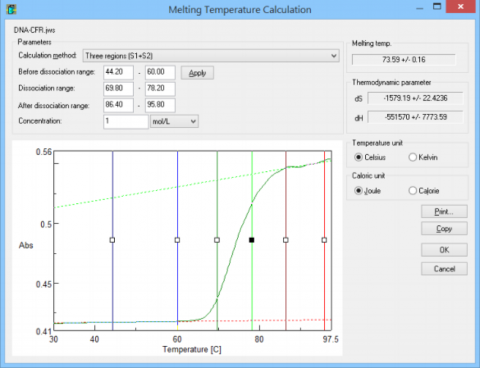# Bio Applications

VRBP-790 | Bio Package
The Bio Package for Spectra Manager Suite and the iRM hand-held controller  include:

Protein Nucleic Acid Measurement
Parallel (Kinetics) Time Course Measurement
Temperature Control Measurement
DNA Melting Analysis

VWTP-959 | Temperature Control Measurement
Perform thermal melting measurement by monitoring changes in the photometric value of samples at a fixed wavelength with change in temperature. The melting temperature can be calculated from the measured temperature-dependent data using the Melting Temperature Calculation program. A peltier thermostatted cell holders is required.VWPN-952 | Protein/Nucleic Acid Measurement
Measure spectra or fixed wavelength of proteins and/or nucleic acids and calculates the sample concentration. Quantitation can be made using one of six fixed methods or a user-defined method.Calculate initial velocity, [V], of enzymatic reactions based on time-course data, and velocity parameters (maximum velocity [Vmax], Michaelis-Menten constant, [Km], and the Hill coefficient, [n]) based on a plot of the initial velocity, [V], versus the substrate concentration [S]. The enzymatic activity can also be determined.

VWTP-780 | DNA Melting AnalysisEstimate the melting temperature of a protein or nucleic acid based on temperature-dependent data using either the Temperature Control Measurement or Temperature Interval Scan Measurement programs. The melting temperature can be calculated using any of the three methods: 2nd derivative, Least squares, and One-point.Melting Temperature Calculation
This program is used for estimating the melting temperature of protein or nucleic acid samples based on temperature-dependent data using either the Temperature Control Measurement or Temperature Interval Scan Measurement programs.